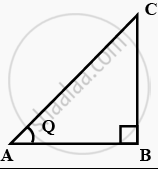# Given sec θ = 13/12 , calculate all other trigonometric ratios. - Mathematics

Sum

Given sec θ = 13/12 , calculate all other trigonometric ratios.

#### Solution

Let ΔABC be a right-angled triangle, right angled at point B.It is given that:

sec θ = hypotenuse / side adjacent to ∠θ = AC/AB = 13/12

Let AC = 13k and AB = 12k, where k is a positive integer.

Applying pythagoras theorem in Δ ABC, we obtain:

AC2 = AB2 + BC2

BC2 = AC2 - AB2

BC2 = (13k)2 - (12k)2

BC2 = 169 k2 - 144 k2

BC2 = 25k2

BC = 5k

BC = 5k

sinθ = ("side opposite to" ∠θ)/("hypotenuse") = ("BC")/("AC") = 5/13

cosθ = ("side adjacent to" ∠θ)/("hypotenuse") = ("AB")/("AC") = 12/13

tanθ = ("side opposite to" ∠θ)/("side adjacent to" ∠θ) = "(BC)"/"(AB)" = 5/12

cotθ = ("side adjacent to" ∠θ)/("side opposite to" ∠θ) = ("AB")/("BC") = 12/5

cosecθ = ("hypotenuse")/("side opposite to" ∠θ) = ("AC")/("BC") = 13/5

Concept: Trigonometric Ratios
Is there an error in this question or solution?
Chapter 8: Introduction to Trigonometry - Exercise 8.1 [Page 181]

#### APPEARS IN

NCERT Class 10 Maths
Chapter 8 Introduction to Trigonometry
Exercise 8.1 | Q 5 | Page 181
Share